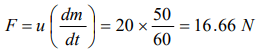## Laws of Motion Questions and Answers Part-6

1. A mass of 10 gm is suspended by a string and the entire system is falling with a uniform acceleration of $400 cm\diagup sec^{2}$  . The tension in the string will be $\left(g=980 cm\diagup sec^{2}\right)$
a) 5,800 dyne
b) 9,800 dyne
c) 11,800 dyne
d) 13,800 dyne

Explanation: T = m(g − a) = 10(980 – 400) = 5800 dyne

2. A second's pendulum is mounted in a rocket. Its period of oscillation decreases when the rocket
a) Comes down with uniform acceleration
b) Moves round the earth in a geostationary orbit
c) Moves up with a uniform velocity
d) Moves up with uniform acceleration

Explanation:3. Two balls of masses $m_{1}$ and $m_{2}$ are separated from each other by a powder charge placed between them. The whole system is at rest on the ground. Suddenly the powder charge explodes and masses are pushed apart. The mass $m_{1}$ travels a distance $s_{1}$ and stops. If the coefficients of friction between the balls and ground are same, the mass $m_{2}$ stops after travelling the distance
a) $s_{2}=\frac{m_{1}}{m_{2}}s_{1}$
b) $s_{2}=\frac{m_{2}}{m_{1}}s_{1}$
c) $s_{2}=\frac{m_1^2}{m_2^2}s_{1}$
d) $s_{2}=\frac{m_2^2}{m_1^2}s_{1}$

Explanation:4. A force vector applied on a mass is represented as $F=6\hat{i}-8\hat{j}+10\hat{k}$    and accelerates with $1 m\diagup s^{2}$ . What will be the mass of the body
a) $10\sqrt{2 } kg$
b) $2\sqrt{10 } kg$
c) 10 kg
d) 20 kg

Explanation:5. A cart of mass M is tied by one end of a massless rope of length 10 m. The other end of the rope is in the hands of a man of mass M. The entire system is on a smooth horizontal surface. The man is at $x=0$ and the cart at $x=10$ m. If the man pulls the cart by the rope, the man and the cart will meet at the point
a) $x=0$
b) $x=5m$
c) $x=10 m$
d) They will never meet

Explanation: In the absence of external force, position of centre of mass remain same therefore they will meet at their centre of mass.

6. A cricket ball of mass 250 g collides with a bat with velocity 10 m/s and returns with the same velocity within 0.01 second. The force acted on bat is
a) 25 N
b) 50 N
c) 250 N
d) 500 N

Explanation:7. A pendulum bob of mass 50 gm is suspended from the ceiling of an elevator. The tension in the string if the elevator goes up with uniform velocity is approximately
a) 0.30 N
b) 0.40 N
c) 0.42 N
d) 0.50 N

Explanation: T = mg = 50 * 10-3 * 10 = 0.5 N

8. A train is moving with velocity 20 m/sec. on this dust is falling at the rate of 50 kg/minute. The extra force required to move this train with constant velocity will be
a) 16.66 N
b) 1000 N
c) 166.6 N
d) 1200 N

Explanation:9. The average force necessary to stop a bullet of mass 20 g moving with a speed of 250 m/s, as it penetrates into the wood for a distance of 12 cm is
a) $2.2\times 10^{3} N$
b) $3.2\times 10^{3} N$
c) $4.2\times 10^{3} N$
d) $5.2\times 10^{3} N$